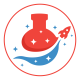# Binary code for kids explainedPosted by

Today, we decided to introduce a binary code to kids to demonstrate how computers save and process the data. We have discussed what a bit and byte is, and what is the binary system.

Imagine trying to describe every scene in a film, every street in your town, or every note in your favorite song using only the numbers 1 and 0.

That’s what your device is doing, using the language of binary code. Computers use binary because it’s a reliable way of storing data.

The genius of this system is that a given binary sequence doesn’t have a predetermined meaning. You can use it to code numbers, letters, pixels and son on.

Let’s take numbers. In usual decimal notation, each digit multiplies by ten and is raised to the value of its position, starting from zero on the right.

Number 43 in decimal form is 4×10¹ + 3×10⁰.

Binary system works similar. A binary number is a number expressed in the base -2 numeral system, which uses only two symbols: zero and one.

This system is a positional notation of a radix of 2. Each digit is a bit.

So how do we present “43” in the binary system? 43 is: 101011

Starting from the right: 1 x 2^5 (which is 32) + 0 x 2^4 (which is 0) + 1 x 2^3 (which is 8) + 0 x 2^2 (which is 0) + 1 x 2^1 (which is 2) + 1 x 2^0 (which is 1), so it will be 43. Number 43 consists of 6 bits of information to encode it.

Once we know what the bit is we can explain that a byte consists of 8 bits.

## How to present a binary code to kids?

Now let’s look at what numbers Atena has drawn!

Let’s agree that red is 1 and blue is 0

She has:

• Red Red Red Red Red Red Blue Red, so it will be 11111101, which is 253
• Red Red Blue Blue Red Red Red Red, so it will be 11001111, which is 207

## What do we need to perform binary code teaching activity?

• An Egg box
• Paint and a brush

## What skills does the binary code activity develop?

• Math
• Computer science – basics

1.Ewa Jurkiewicz says:
1.Atena SciAndPlay says: